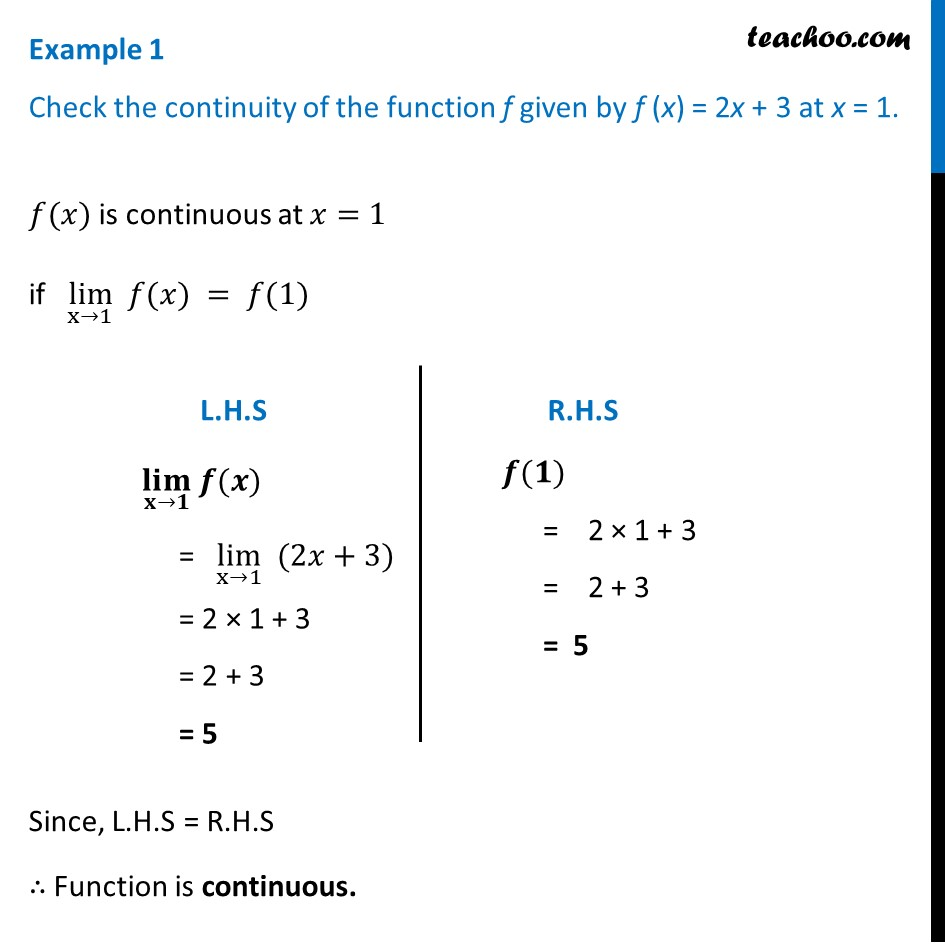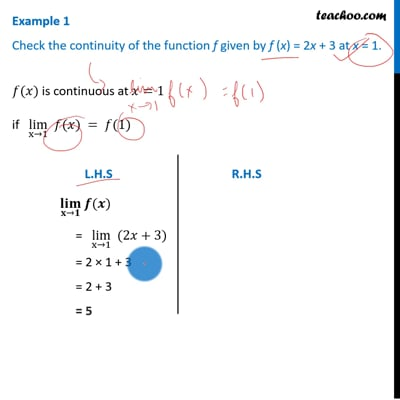Checking continuity at a given point

Chapter 5 Class 12 Continuity and Differentiability
Concept wiseThis video is only available for Teachoo black users

Introducing your new favourite teacher - Teachoo Black, at only ₹83 per month

### Transcript

Example 1 Check the continuity of the function f given by f (x) = 2x + 3 at x = 1. 𝑓(𝑥) is continuous at 𝑥=1 if lim┬(x→1) 𝑓(𝑥) = 𝑓(1) Since, L.H.S = R.H.S ∴ Function is continuous. (𝐥𝐢𝐦)┬(𝐱→𝟏) 𝒇(𝒙) "= " lim┬(x→1) " "(2𝑥+3) = 2 × 1 + 3 = 2 + 3 = 5 𝒇(𝟏) = 2 × 1 + 3 = 2 + 3 = 5